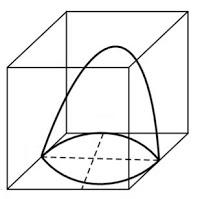# NCERT Solutions Class 10 Mathematics Solutions for Surface Areas and Volumes - Exercise 13.1 in Chapter 13 - Surface Areas and Volumes

A hemispherical depression is cut out from one face of a cubical wooden block such that the diameter l of the hemisphere is equal to the edge of the cube. Determine the surface area of the remaining solid.

The diagram is as follows:Now, the diameter of hemisphere = Edge of the cube = l

So, the radius of hemisphere = l/2

∴ The total surface area of solid = surface area of cube + CSA of hemisphere – Area of base of hemisphere

TSA of remaining solid = 6 (edge)2+2πr2-πr2

= 6l^2+ πr^2\\ = 6l^2+π(\frac{l}{2})^2\\ = 6l^2+π\frac{l^2}{4}\\ = \frac{l^2}{4}(24+π)\ \text{sq. units}

Related Questions

Lido

Courses

Teachers

Book a Demo with us

Syllabus

Maths
CBSE
Maths
ICSE
Science
CBSE

Science
ICSE
English
CBSE
English
ICSE
Coding

Terms & Policies

Selina Question Bank

Maths
Physics
Biology

Allied Question Bank

Chemistry
Connect with us on social media!